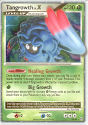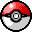Caitlyn's Pokémon Card Collection

CardType=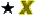LV. X

21 records

 LV. X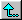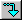Alakazam 4 LV. X  LV. X   HolographicCharacter = Alakazam   Card Set = Platinum - Rising Rivals   Card # in Set = 103 / 111   Country = USA   Year = 2009   Illustrator = Ryo Ueda   Rarity = Rare Holo   HP Value = 100   Evolution Stage = Stage 2   Region = Kanto   Quantity = 1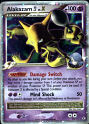Arceus LV. X  LV. X   HolographicCharacter = Arceus   Card Set = Platinum - Arceus   Card # in Set = 94 / 99   Country = USA   Year = 2009   Illustrator = Shizurow   Rarity = Rare Holo   HP Value = 120   Evolution Stage = Basic   Region = Sinnoh   Quantity = 1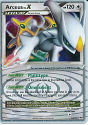Arceus LV. X (comments)  LV. X   Holographic   PromoCharacter = Arceus   Card Set = DP Promos   Card # in Set = DP53   Country = USA   Year = 2009   Illustrator = Shizurow   Rarity = Rare Holo   HP Value = 120   Evolution Stage = Basic   Region = Sinnoh   Caitlyn's rating = 3 (good)   Quantity = 1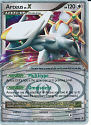Empoleon LV. X  LV. X   Holographic   PromoCharacter = Empoleon   Card Set = DP Promos   Card # in Set = DP11   Country = USA   Year = 2007   Illustrator = Shizurow   HP Value = 140   Evolution Stage = Stage 2   Region = Sinnoh   Quantity = 1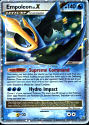Floatzel GL LV. X  LV. X   HolographicCharacter = Floatzel   Card Set = Platinum - Rising Rivals   Card # in Set = 104 / 111   Country = USA   Year = 2009   Illustrator = Mitsuhiro Arita   Rarity = Rare Holo   HP Value = 100   Evolution Stage = Stage 1   Region = Sinnoh   Quantity = 1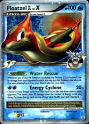Flygon LV. X  LV. X   HolographicCharacter = Flygon   Card Set = Platinum - Rising Rivals   Card # in Set = 105 / 111   Country = USA   Year = 2009   Illustrator = Shizurow   Rarity = Rare Holo   HP Value = 140   Evolution Stage = Stage 2   Region = Hoenn   Quantity = 1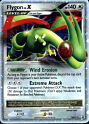\$B%U!=%G%#%s;M(B LV.X Fuudin 4 LV.X (Alakazam 4 LV.X)  LV. X   First EditionCharacter = Alakazam   Card Set = Platinum 2 (Japanese Pt2)   Card # in Set = 42 / 90   Country = Japan   Year = 2008   Illustrator = Ryo Ueda   Rarity = Rare Holo   HP Value = 100   Evolution Stage = Stage 2   Region = Kanto   Caitlyn's rating = 4 (excellent)   Quantity = 1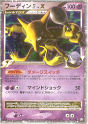Gallade 4 LV. X  LV. X   HolographicCharacter = Gallade   Card Set = Platinum - Rising Rivals   Card # in Set = 106 / 111   Country = USA   Year = 2009   Illustrator = Ryo Ueda   Rarity = Rare Holo   HP Value = 100   Evolution Stage = Stage 2   Region = Sinnoh   Quantity = 1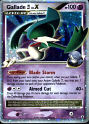Gardevoir LV. X  LV. X   HolographicCharacter = Gardevoir   Card Set = DP - Secret Wonders   Card # in Set = 131 / 132   Country = USA   Year = 2007   Illustrator = Ryo Ueda   Rarity = Rare Holo   HP Value = 130   Evolution Stage = Stage 2   Region = Hoenn   Quantity = 1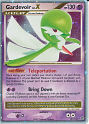Giratina LV. X (comments)  LV. X   Holographic   PromoCharacter = Giratina   Card Set = DP Promos   Card # in Set = DP38   Country = USA   Year = 2009   Illustrator = Shizurow   Rarity = Rare Holo   HP Value = 130   Evolution Stage = Basic   Region = Sinnoh   Caitlyn's rating = 4 (excellent)   Quantity = 1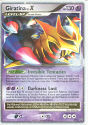Hippowdon LV. X  LV. X   HolographicCharacter = Hippowdon   Card Set = Platinum - Rising Rivals   Card # in Set = 107 / 111   Country = USA   Year = 2009   Illustrator = Shizurow   Rarity = Rare Holo   HP Value = 130   Evolution Stage = Stage 1   Region = Sinnoh   Quantity = 1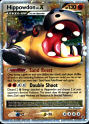Infernape 4 LV. X  LV. X   HolographicCharacter = Infernape   Card Set = Platinum - Rising Rivals   Card # in Set = 108 / 111   Country = USA   Year = 2009   Illustrator = Ryo Ueda   Rarity = Rare Holo   HP Value = 110   Evolution Stage = Stage 2   Region = Sinnoh   Quantity = 1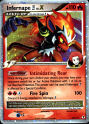Lucario LV. X  LV. X   HolographicCharacter = Lucario   Card Set = DP - Mysterious Treasures   Card # in Set = 122 / 123   Country = USA   Year = 2007   Illustrator = Ryo Ueda   Rarity = Rare Holo   HP Value = 110   Evolution Stage = Basic   Region = Sinnoh   Quantity = 1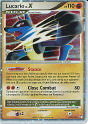Luxray GL LV. X  LV. X   HolographicCharacter = Luxray   Card Set = Platinum - Rising Rivals   Card # in Set = 109 / 111   Country = USA   Year = 2009   Illustrator = Mitsuhiro Arita   Rarity = Rare Holo   HP Value = 110   Evolution Stage = Stage 2   Region = Sinnoh   Quantity = 1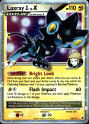Machamp LV. X  LV. X   HolographicCharacter = Machamp   Card Set = DP - Stormfront   Card # in Set = 98 / 100   Country = USA   Year = 2008   Illustrator = Ryo Ueda   Rarity = Rare Holo   HP Value = 150   Evolution Stage = Stage 2   Region = Kanto   Quantity = 1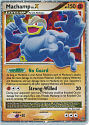Mismagius GL LV. X  LV. X   HolographicCharacter = Mismagius   Card Set = Platinum - Rising Rivals   Card # in Set = 110 / 111   Country = USA   Year = 2009   Illustrator = Kent Kanetsuna   Rarity = Rare Holo   HP Value = 100   Evolution Stage = Stage 1   Region = Sinnoh   Quantity = 1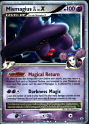\$B%`%&%^!<%8(BGL LV. X Muumaaji GL LV. X (Mismagius GL LV. X)  LV. X   Holographic   PromoCharacter = Mismagius   Card Set = DPt-P Promo   Card # in Set = 11 / 51   Country = Japan   Illustrator = Kent Kanetsuna   Rarity = Rare Holo   HP Value = 100   Evolution Stage = Stage 1   Region = Sinnoh   Quantity = 1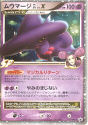Rayquaza C LV. X  LV. X   Holographic   PromoCharacter = Rayquaza   Card Set = DP Promos   Card # in Set = DP47   Country = USA   Year = 2009   Illustrator = Shizurow   Rarity = Rare Holo   HP Value = 120   Evolution Stage = Basic   Region = Hoenn   Quantity = 1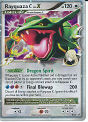Shaymin LV. X  LV. X   Holographic   PromoCharacter = Shaymin   Card Set = DP Promos   Card # in Set = DP39   Country = USA   Year = 2009   Illustrator = Shizurow   Rarity = Rare Holo   HP Value = 110   Evolution Stage = Basic   Region = Sinnoh   Quantity = 1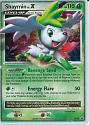Snorlax LV. X  LV. X   HolographicCharacter = Snorlax   Card Set = Platinum - Rising Rivals   Card # in Set = 111 / 111   Country = USA   Year = 2009   Illustrator = Shizurow   Rarity = Rare Holo   HP Value = 130   Evolution Stage = Basic   Region = Kanto   Quantity = 1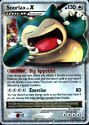Tangrowth LV. X  LV. X   HolographicCharacter = Tangrowth   Card Set = Platinum - Arceus   Card # in Set = 99 / 99   Country = USA   Year = 2009   Illustrator = Ryo Ueda   Rarity = Rare Holo   HP Value = 130   Evolution Stage = Stage 2   Region = Sinnoh   Quantity = 1